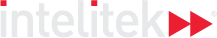### Course Overview

Algebra 1 begins with a review of the fundamental concepts necessary for the study of algebra, among them exponents, monomials and polynomials. We then move on to working with algebraic fractions and factorization, concluding with solving linear equations. Special emphasis is given to “translating” word statements into algebraic expressions and equations.

HOURS OF INSTRUCTION: 15

### Course Outline

• Getting Started with Algebra
• Operations on Monomials and Polynomials
• Algebraic Fractions
• Factorization
• Solving Linear Equations